# Create charts

To create interactive charts, select one or more string, number, rate/ratio, or date/time fields from the data pane and drag them onto the page, or use the Chart button at the top of the data pane.

You can also switch to different supported visualization types from existing cards.

##### Tip: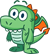You can copy a chart card onto another page by dragging it to the New page tab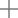or an already created page. If you want to copy the chart onto the same page, use Ctrl+C to copy and Ctrl+V to paste.

## Chart types

There are several types of charts that can be created using ArcGIS Insights. Insights uses smart defaults to create the best type of chart for the data you want to visualize, so you know you are always getting the best type of chart for the field that you select.

The table below indicates the inputs for each chart, related visualizations you can switch to using the Visualization type button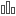, and questions the chart can help you answer.

VisualizationMinimum data requirementsRelated visualization typesQuestions it can help you answer

Bar chart

One string field

• Unique values map
• Summary table
• Reference table
• KPI
• Column chart
• Treemap
• Bubble chart
• Line graph
• Donut chart
• Point chart

How is it distributed (by category)?

Box plot

One number or rate/ratio field

• Proportional symbol map
• Summary table
• Reference table
• KPI
• Histogram

How is it distributed?

What are the high values?

What are the low values?

Bubble chart

One string field

• Unique values map
• Summary table
• Reference table
• KPI
• Bar chart
• Column chart
• Treemap
• Line graph
• Donut chart

How is it distributed (by category)?

Chord diagram

Two string fields

• Summary table
• Reference table
• Bar chart
• Stacked bar chart
• Column chart
• Stacked column chart
• Treemap
• Heat chart
• Data clock
• Line graph

How is it related?

Column chart

One string field

• Unique values map
• Summary table
• Reference table
• KPI
• Bar chart
• Treemap
• Bubble chart
• Line graph
• Donut chart
• Point chart

How is it distributed (by category)?

Combo chart

One of the following:

• One string field and two number or rate/ratio fields
• Two datasets with the same string field
• Summary table
• Reference table
• Treemap
• Scatter plot
• Point chart

How is it distributed (by category)?

How is it related?

Data clock

Two string fields

• Summary table
• Reference table
• Bar chart
• Stacked bar chart
• Column chart
• Stacked column chart
• Treemap
• Heat chart
• Chord diagram
• Line graph

How has it changed?

How is it related?

Donut chart

One string field

• Unique values map
• Summary table
• Reference table
• KPI
• Bar chart
• Column chart
• Treemap
• Bubble chart
• Line graph

How is it distributed (by category)?

Heat chart

Two string fields

• Summary table
• Reference table
• Bar chart
• Stacked bar chart
• Column chart
• Stacked column chart
• Treemap
• Chord diagram
• Data clock
• Line graph

How is it related?

Histogram

One number field

• Proportional symbol map
• Summary table
• Reference table
• KPI
• Box plot

How is it distributed?

What are the high values?

What are the low values?

KPI

One number field

None

How is it related?

Line graph

One string field

• Unique values map
• Summary table
• Reference table
• KPI
• Bar chart
• Column chart
• Treemap
• Bubble chart
• Donut chart

How is it distributed (by category)?

Two string fields

• Summary table
• Reference table
• Bar chart
• Stacked bar chart
• Column chart
• Stacked column chart
• Treemap
• Heat chart
• Chord diagram
• Data clock
• Line graph

How is it related?

Point chart

One string field and one number or rate/ratio field

• Summary table
• Reference table
• KPI
• Bar chart
• Column chart
• Treemap
• Bubble chart
• Line graph
• Box plot
• Donut chart

How is it distributed (by category)?

Scatter plot

Two number or rate/ratio fields

• Summary table
• Reference table
• KPI

How is it related?

Scatter plot matrix

Three or more number or rate/ratio fields

• Summary table
• Reference table

How is it related?

Stacked bar chart

Two string fields

• Summary table
• Reference table
• Bar chart
• Column chart
• Stacked column chart
• Treemap
• Heat chart
• Chord diagram
• Data clock
• Line graph

How is it distributed (by category)?

Stacked column chart

Two string fields

• Summary table
• Reference table
• Bar chart
• Stacked bar chart
• Column chart
• Treemap
• Heat chart
• Chord diagram
• Data clock
• Line graph

How is it distributed (by category)?

Time series graph

One date/time field

• Reference table

How has it changed?

Treemap

One string field

• Unique values map
• Summary table
• Reference table
• KPI
• Bar chart
• Stacked bar chart
• Column chart
• Stacked column chart
• Heat chart
• Chord diagram
• Data clock
• Line graph
• Point chart

How is it distributed (by category)?

## Change variables

As the data story emerges from your visualizations, you can show different data and adjust properties such as grouping your data, as well as change chart styling. Editable fields on charts are shown in gray drop-down boxes. You can choose a different field to show on one axis or both axes. Click an editable field on your chart, and choose a different field from the list.

Visualizations that summarize data, such as bar charts, time series graphs, line graphs, and summary tables allow you to choose a different statistic type. For example, you can switch from displaying the sum of sales per region to the average sales per region by using the statistic type drop-down menu.

## Use the chart toolbar

Every card has a toolbar that can be used to find more information or improve the appearance of your data.

ToolDescriptionApplicable charts

Layer options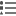The Layer options button can be used to display the Layer options pane. The options available on this pane depend on the chart type.

All

Chart statistics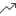The Chart statistics button can be used to display relevant statistical calculations about your data. The following statistics can be displayed for bar charts, column charts, time series graphs, and line graphs:

• Mean
• Median
• Upper quartile
• Lower quartile
• Custom

The following statistics can be displayed for histograms:

• Mean
• Median
• Normal distribution

The following statistics can be displayed for scatter plots:

• None
• Linear
• Exponential
• Polynomial

Bar chart, column chart, histogram, scatter plot, time series graph, line graph

Card filter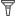The Card filter button can be used to remove any unwanted data from your chart. Filters can be applied to all string, number, rate/ratio, and date/time fields. A card filter does not affect other cards using the same dataset.

All

Selection tools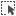Selections can be used to highlight important features. Selections on one card are reflected on any other card using the same data. The following selection tools may be available on your chart:

• Select
• Box select
• Invert selection

All except donut chart and KPI

Visualization typeThe Visualization type drop-down menu shows all available card types. The drop-down list can be used to quickly change your card to a map, summary table, or another type of card. The availability of visualizations depends on what type of data is being displayed on your card.

All

Sort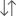The Sort option is available for most charts that use a string field. The following sort options are available:

• Sort ascending
• Sort descending
• Sort A to Z
• Sort Z to A
• Sort manually (available with bar and column charts)

Bar chart, column chart, line graph

Maximize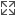The Maximize button is used to enlarge the card. Other cards on the page will be reduced to thumbnails. The card can be returned to its previous size using the Restore down button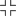.

All

Enable cross filters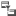The Enable cross filters button is used to allow filters to be created on the card using selections on other cards. Cross filters can be removed using the Disable cross filters button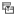.

All

Flip card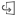The Flip card button displays information on the back of the card about your chart, such as a description of your analysis and a time stamp for when your data was last updated, if applicable. The back of the card also includes statistics, such as count, mean, and median.

For some charts, the back of the card can be used to export the data from the card as a CSV.

All

Delete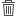The Delete button removes the card from your page. If you did not intend to delete your card, you can retrieve it using the Undo button.

All

## Modify an axis

By default, all numeric axes in Insights are on a linear scale. One or more axis can be changed to a logarithmic scale for scatter plots, time series graphs, and line charts. Logarithmic scales are nonlinear scales that can be used to display datasets with large ranges of data values. A logarithmic scale uses logarithmic orders of magnitude to display the data at a more manageable scale. By default, Insights will use a base 10 logarithmic scale. Changing the scale of the axis does not change the value of the data, just the way it is displayed.

The scale of an axis can be changed by clicking the axis and choosing Linear or Log.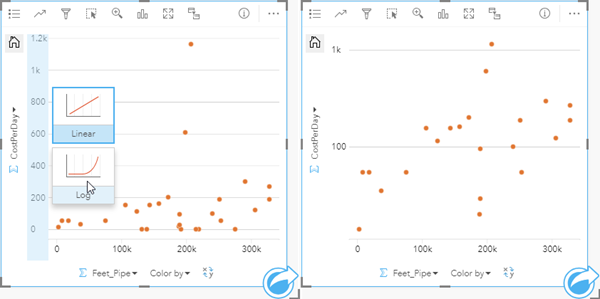Left—Changing the scale of the y-axis to Log by clicking the axis and choosing the Log scale. Right—A scatter plot with the x-axis displaying data on a linear scale and the y-axis displaying data on a logarithmic scale.

Field names are used to label axes by default. To change the axis labels on a chart, use the following steps:

1. Click the Card options button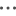.
2. Click the Edit labels button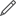.
3. Click a label to highlight the text.
4. Type the new axis label.
5. Hit Enter or Return on your keyboard to make the changes go into effect.

## Change the layer options

The Layer options pane can be accessed by clicking the Layer options button. The options and tabs available in the Layer options pane are dependent on the type of chart and current style.

TabDescriptionApplicable charts

LegendThe Legend tab displays the symbols on the chart along with the corresponding categories. The Legend tab is interactive, so it can be used to select features on your chart (available for unique symbols).

If your chart is displayed using Unique symbols, you can change the color associated with a category by clicking the symbol and choosing a color from the palette or entering a hex value.

All except histograms

Symbology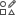The Symbology tab is used for the following purposes:

• Bar and column charts — Change the Symbol type between Single symbol and Unique symbols. Other charts that can be symbolized using unique or single symbols, such as scatter plots, must be changed using Subgroup or Color by on the x- or y-axis.
• Bar, column, line, and combo charts — Add value labels to the chart.
• Data clocks and heat charts — Change the Classification type between natural breaks, equal interval, or quantile classifications.
• Link charts — Change the layout of the chart between Force directed, Hierarchical, and Radial.
• Point charts — Add confidence intervals to your chart.

Bar chart, column chart, combo chart, data clock, heat chart, link chart, point chart

Appearance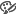The Appearance tab can be used to change the Symbol color for charts displayed with single symbols, such as histograms. The Symbol color can be chosen from the color palette or customized using a hex code.

Bar chart, column chart, combo chart, histogram, scatter plot, time series graph, line graph, box plot, scatter plot matrix, link chart, point chart

## Copy a chart

A chart card can be duplicated on a page by activating the card (a card is active when the Action button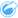is visible) and using Ctrl+C to copy the card and Ctrl+V to paste it on the page.

A chart card can be copied to a different page by dragging the card to the New page tabor to an existing page. When a chart is copied to a new page, the dataset will also be copied, if necessary, along with all of the processes used to create the chart. The dataset will not be copied if it already exists on the new page unless one of the datasets contains a dataset filter, a calculated field, or a location field created by enabling location.